# What is the density of the room

D.-E. Liebscher, http://www.aip.de/~lie/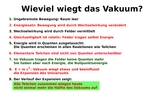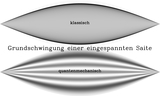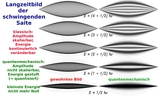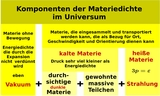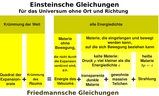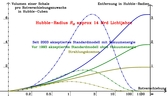An absurd question, it seems. But if one follows the findings of the physics of the 20th century, it becomes inevitable to understand vacuum as the pure absence of preparable and identifiable objects, molecules, atoms, particles, quanta, but not as the absence of energy. But energy carries mass (we know that in the formula E = mc2), and this should add gravity. We want to understand how much it is.

Energy is transferred in quanta and the transfer always looks like a collision of mechanical particles. All elementary particles can and are therefore understood as quanta of different fields. In the vacuum there is no longer any transferable energy, but that does not rule out the possibility that there is still energy that is noticeable through its gravitational effect. It is determined by this gravity in the universe. How much does the energy of empty space contribute?

The answer is a conclusion that begins by stating that movement must first take place in an empty space. If the room is filled with something, like our surroundings with the air, then it has to move to the side if a body wants to move through it. We see that in the air resistance, among other things. In the empty space the movement does not change at first, it is straight and uniform. Changing this movement requires interaction with other bodies, which paradoxically now seems to bridge the empty space and enables the exchange of energy, momentum and other variables that can be balanced. We speak of force fields when we mean this.

But space and time have a strange geometry, which is examined in the theory of relativity. We only have to know here that simultaneity cannot be determined well over the entire space, and with interacting bodies the balance of their impulses and energies just rises when we also ascribe energy and momentum to the fields themselves. The electromagnetic field carries energy, which we feel in the thermal radiation, and its impulse can be elementarily detected in the light pressure.

Now, while studying atomic systems, we have learned that energy can only be transferred from one object to another in small quanta. These quanta are so small by the standards of everyday life that we cannot perceive them individually, but they are essential in the exchange of energy in atomic systems. It now turns out that we can understand the fields as the sum of such quanta, which in turn behave like particles. Conversely, the elementary particles have to be represented as quanta of corresponding fields. This is not crazy, but has led to important discoveries, such as that of antiparticles.

So on a small scale, the world is filled with fields, the quanta of which move like particles and interact with one another. Vacuum is the absence of all these particles, the absence of energy that can be absorbed as quanta.

However, an atomic system is not at rest even if the account of the withdrawable energy is empty. A residual movement, the so-called zero point fluctuations, remains, and calculating its energy as a whole for all essential fields is not a simple problem, nor is it finally solved. We only know that the vacuum, if its energy density is different from zero, cannot change it by changing the volume it occupies. The pressure of the vacuum must be negative and as great as the energy density itself.

Where can we observe the suspected severity of the vacuum? Certainly not on objects that can be isolated, because the vacuum cannot be isolated at the moment, and neither is it in their surroundings. We feel the weight of the vacuum only in the course of the expansion of the universe, which is determined by the gravity of all its components. We measure this course with the methods of astronomy, and we see today that the vacuum has a constant density and that this already far outweighs the density of the particles (which is constantly thinning in the expanding universe) (vacuum to particles roughly like 7: 3). The empty space is already more than twice as heavy as its entire contents.

Dierck-E. Liebscher, Astrophysical Institute Potsdam, http://www.aip.de/~lie/